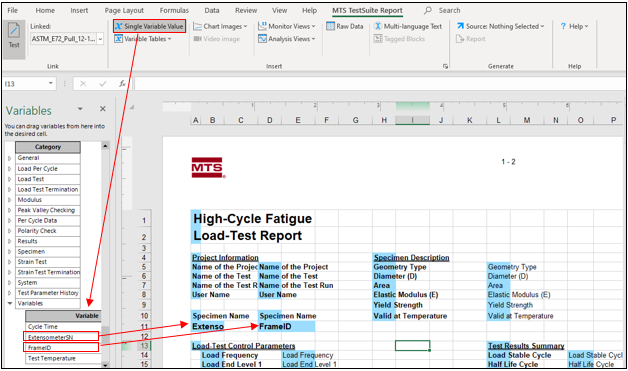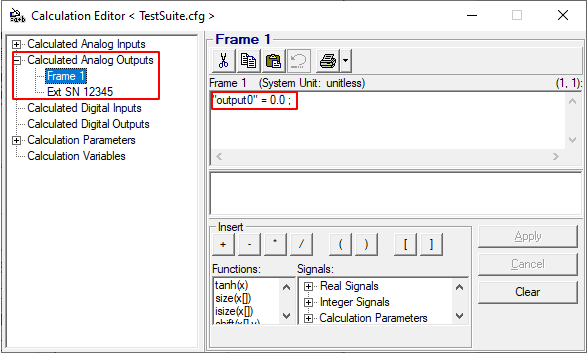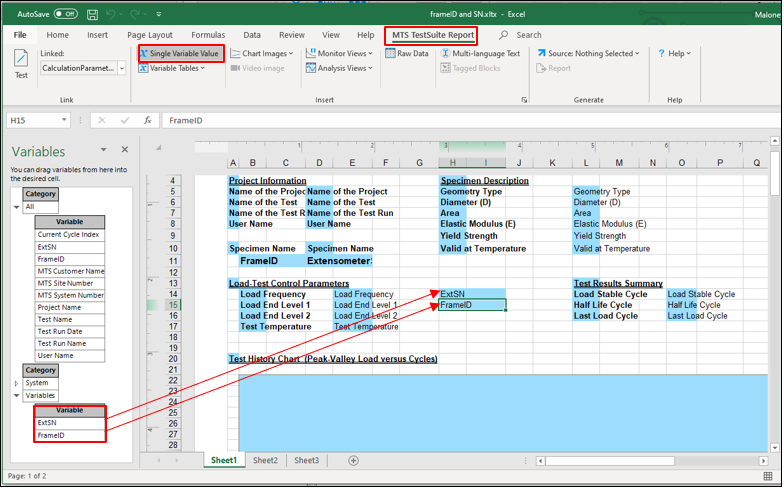This content is available if you have a software support plan or current system warranty. To request access to this content, please submit a support request here.

# How do I add additional information like Frame ID and Extensometer serial number to my report template

QUESTION

How do I add additional information like Frame ID and Extensometer serial number to my report template?

Go to the Variables tab and click the plus sign (+) to create a new variable called ExtensometerSN or something to identify that it's the extensometer serial number and enter the SN under the Properties tab (Figure 1).  Do the same for FrameID and any other things you want to add to the report template later.Figure 1 – Creating new variables in TestSuite

You can add an Input Variables activity near the start of your test procedure to prompt the user to enter values for the variables if they will change with each test run (Figure 2).  If they will not change you can just enter the values as default parameters in the Properties window under the variable instead.Figure 2 -  Adding an Input Variables activity to the test procedure

Save the test once the new variables have been added.

Open your report template and make sure you are linked to the test.

Click on the Single Variable Value button under the MTS TestSuite Report tab (Figure 3).

Expand the Variables node until you find your new variables.  Drop and drag them to where you want them in the report template and save the changes (Figure 3).Figure 3 – Adding new variables to a report template

Method 2

There is a way to bring in the variable value directly from Station Manager.  This method is good if you have more than one station with unique parameters allowing you to directly import them from the station connected to TestSuite.

Create a Calculated Output in Station Builder named after the variable values, i.e., Frame number and Extensometer serial number (Figure 1).  Dimension and Display Units should be set to unitless.

This method requires the user to have the 793.03 Calculations with Outputs Option.  This option gets installed with an additional keycode at the time of the 793 System Software installation.Figure 1 – Creating Calculated Outputs in Station Builder

The Calculated Analog Outputs will appear in the Calculations Editor (Figure 2).  Leave the calculations as is. That way the value that gets imported to TestsSuite later will be the name of the Calculated Output you created in Station Builder which should reflect the frame’s name and transducer’s serial number.Figure 2 – Calculation Editor Calculated Analog Outputs

Open TestSuite and connect to the open station in Station Manager.

Go to the Resources tab > Import Resources > Import All Unused Controller Resources

This will bring in the calculated analog outputs from Station Manager under the Float Signals list (Figure 3)Figure 3 – Imported Resources from Station Manager to TestSuite

Go to the Variables tab and click the (+) sign to add new variables.  Name them after the calculated analog outputs from Station Manager (Figure 4)..

Click the Is Calculated check box and set the Type as Text.

Use the Signals/Channels list to set the calculation equal to the calculated analog output you imported from Station Manager.Figure 4 – Creating a calculated variable equal to the calculated analog output from Station Manager

Add a Calculate Variables activity somewhere in your test flow and add variables you want calculated to the Variables List (Figure 5).Figure 5 – Calculate Variables activity

The variable values should show up in the Variables Summary tab under each test run (Figure 6).Figure 6 – Variable Summary

Open your report template and make sure you are linked to the test.

Click on the Single Variable Value button under the MTS TestSuite Report tab (Figure 7).

Expand the Variables node until you find your new variables.   Drop and drag them to where you want them in the report template and save the changes (Figure 7).Figure 7 – Adding Single Variable Values to the report template

Method 3

The following method works for numerical values only; not text.

Create a Calculation Parameter in the Calculation Editor.  Set the Range to (unitless) and enter the value in Current Value (Figure 1).Figure 1 – Creating Calculation Parameters

Open TestSuite and connect to the open station in Station Manager.

Go to the Resources tab > Import Resources > Import All Unused Controller Resources

This will bring in the Calculation Parameters from Station Manager into TestSuite (Figure 2)Figure 2 – Importing Calculation Parameters into TestSuite

Create a calculated variable and use the CalculationParameter function in the Calculation Editor to calculate the variable based on the imported calculaltion parameter (Figure 3).Figure 3 – Calculated variable using the CalculationParameter function

Open your report template and make sure you are linked to the test.

Click on the Single Variable Value button under the MTS TestSuite Report tab (Figure 4).

Expand the Variables node until you find your new variables.  Drop and drag them to where you want them in the report template and save the changes (Figure 4).Figure 4 – Adding a Single Variable Value to the report template

K
Keary is the author of this solution article.

Did you find it helpful? Yes No

Send feedback